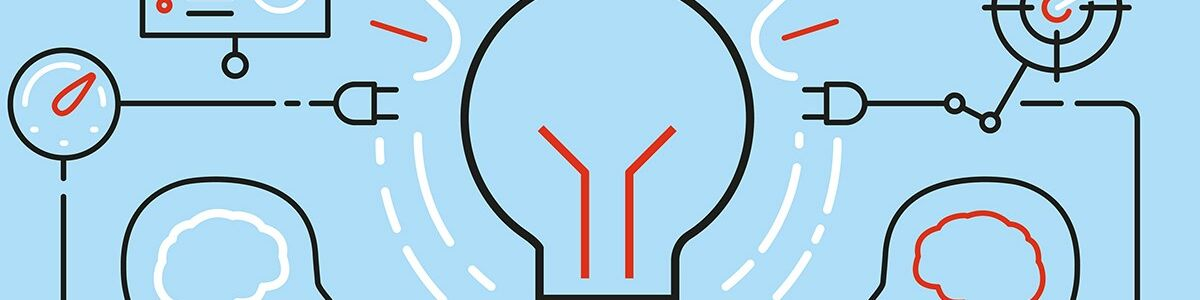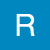Updated by rogersnancy2020 on Jul 21, 2020REPORTrogersnancy2020
Owner
10 items   1 followers   0 votes   4 views

# Top 10 Machine Leaning Algorithm in 2020 You Can Not Miss to Become a Data Scientist!

This is a list of most important machine learning algorithms in 2020. If you are a data scientist/ML engineer or want to be a data scientist/ML engineer, you must learn these algorithms. Please check the list and provide any suggestions you think good to make a great list. Don't forget to vote and share!

1

## Support Vector Machine

Support Vector Machine is a discriminative classifier which finds the optimal hyperplane that distinctly classifies the data points in an N-dimensional space(N - the number of features). In a two dimensional space, a hyperplane is a line that optimally divides the data points into two different classes.

This algorithm is widely applied in classification and regression problems.

Get a detailed idea here!

2

## Decision Tree Algorithm

This algorithm is based on a decision tree structure. A decision tree is a form of a tree or hierarchical structure that breaks down a dataset into smaller and smaller subsets. At the same time, an associated decision tree is incrementally developed. The tree contains decision nodes and leaf nodes. The decision nodes(e.g. Outlook) are those nodes represent the value of the input variable(x). It has two or more than two branches(e.g. Sunny, Overcast, Rainy). The leaf nodes(e.g. Hours Played) contains the decision or the output variable(y). The decision node that corresponds to the best predictor becomes the topmost node and called the root node.

This algorithm is also popularly used in both classification and regression problems.

Get a detailed idea [here](https://www.aionlinecourse.com/tutorial/machine-learning/decision-tree-intuition)

3

## Random Forest Algorithm

Random Forest is a supervised learning algorithm. It uses the ensemble learning technique(Ensemble learning is using multiple algorithms at a time or a single algorithm multiple times to make a model more powerful) to build several decision trees at random data points. Then their predictions are averaged. Taking the average value of predictions made by several decision trees is usually better than that of a single decision tree.

It has uses in both regression and classification problems.

Get the detailed algorithm here!

4

## Logistic Regression Algorithm

Logistic Regression is the appropriate regression analysis to solve binary classification problems( problems with two class values yes/no or 0/1). This algorithm analyzes the relationship between a dependent and independent variable and estimates the probability of an event occurring. Like other regression models, it is also a predictive model. But there is a slight difference. While other regression models provide continuous output, Logistic Regression is used to model the probability of a certain class or event existing such as pass/fail, win/lose, alive/dead, or healthy/sick.

Though it is named as a regression algorithm, it is actually used in classification problems.

Get the details here!

5

## K-nearest Neighbor Algorithm

K-nearest neighbor is a non-parametric lazy learning algorithm, used for both classification and regression. KNN stores all available cases and classifies new cases based on a similarity measure. The KNN algorithm assumes that similar things exist in close proximity. In other words, similar things are near to each other. When a new situation occurs, it scans through all past experiences and looks up the k closest experiences. Those experiences (or: data points) are what we call the k nearest neighbors.

This algorithm is best suited for solving classification problems.

Get more details here!

6

## K-means Clustering Algorithm

K-means clustering is an unsupervised algorithm. This algorithm categorizes data points into a predefined number of groups K, where each data point belongs to the group or cluster with the nearest mean. Data points are clustered based on similarities in their features. The algorithm works iteratively to assign each data point to one of the K groups in such a way that the distance(i.e. Euclidian or Manhattan) between the centroid(i.e. the center of the cluster) of that group and the data point be small. The algorithm produces exactly K different clusters of the greatest possible distinction.

This algorithm is mostly used in finding clusters among a set of unlabelled data.

Get the details here!

7

## Naive Bayes Algorithm

It is a classification technique based on Bayes Theorem. In simple terms, it is a probabilistic classifier which assumes that the presence of a particular feature in a class is not related to the presence of other features. It calculates the Posterior probability of all the events using the Bayes Theorem. Then it takes the event that has the maximum posterior probability.

Naive Bayes is effective for the classification of text data and widely used in text mining.

Get the details here!

8

## Upper Confidence Bound Algorithm

This is a reinforcement learning algorithm which is mostly used in solving a special kind of problem called the multi-armed bandit problem.

This is a use case of reinforcement learning, where we are given a slot machine called multi-armed bandit( the slot machines in casinos are called bandit as it turns out all casinos configure these machines in such a way that all gamblers end up losing money!) with each arm having its own rigged probability distribution of success. Pulling any one of these arms gives you a stochastic reward of either 1, for success or 0, for a failure. Now your task is to find such an optimal strategy that will give you the highest rewards in the long run without the prior knowledge of the probability distribution of success of the machines.

This problem can be related to more business examples such as displaying the optimal ads to the viewer.

Upper Confidence Bound is an ideal algorithm to solve such kind of problems.

Get more details here!

9

## XGBoost Algorithm

XGBoost stands for Extreme Gradient Boosting. XGBoost is a decision-tree-based ensemble Machine Learning algorithm that uses a gradient boosting framework. In prediction problems involving unstructured data (images, text, etc.) artificial neural networks tend to outperform all other algorithms or frameworks. However, when it comes to small-to-medium structured/tabular data, decision tree-based algorithms are considered best-in-class right now. XGBoost has been gaining popularity for being a more robust algorithm in terms of performance.

It can be applied both for regression and classification problems.

Get the details here!

10

## Principal Component Analysis Algorithm

Principal Component Analysis or PCA is a popular dimensionality reduction technique that reduces the number of features or independent variables by extracting those features with the highest variance. That means it finds the correlation between the independent variables and calculates their variance, then it selects those features that have the highest variance.

This algorithm is used for extracting the most important features from a higher number of features.

Get the details here!